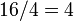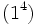# Subgroups of order 4 in groups of order 16

This article contains summary information about all subgroups of order 4 inside groups of order 16. See also groups of order 16 | groups of order 4 | subgroup structure of groups of order 16 | supergroups of groups of order 4

This article describes the occurrence of groups of order 4 as subgroups inside groups of order 16.

There are two groups of order 4: cyclic group:Z4 and Klein four-group. There are 14 groups of order 16.

Note the following:

• A subgroup of order 4 in a group of order 16 is also a subgroup of index 4, because$16/4 = 4$.
• A subgroup of order 4 in a group of order 16 need not be a normal subgroup. However, it has subnormal depth at most 2 so even if it is not normal, it is a 2-subnormal subgroup. If it is not normal, its normal core is a subgroup of order 2 and index 8, and its normal closure is a subgroup of order 8 and index 2.
• There are a few cases where there are multiple automorphism classes of isomorphic subgroups of order 4 in a given group of order 16.

## Numerical information on counts of subgroups

The table below presents information on counts of subgroups of order 4 in groups of order 16. Note the following:

General assertion Implication for the counts in this case
congruence condition on number of subgroups of given prime power order The number of subgroups of order 4 is odd.
The number of normal subgroups of order 4 is odd.
The number of 2-core-automorphism-invariant subgroups of order 4 is odd.
Group Second part of GAP ID Hall-Senior number Hall-Senior symbol Nilpotency class Minimum size of generating set Number of subgroups of order 4
(must be odd)
Number of normal subgroups of order 4
(must be odd)
Number of 2-core-automorphism-invariant subgroups of order 4
(must be odd)
Number of 2-automorphism-invariant subgroups of order 4 Number of characteristic subgroups of order 4
cyclic group:Z16 1 5$(4)$ 1 1 1 1 1 1 1
direct product of Z4 and Z4 2 3$(2^2)$ 1 2 7 7 1 1 1
SmallGroup(16,3) 3 9$16\Gamma_2c_1$ 2 2 11 3 1 1 1
nontrivial semidirect product of Z4 and Z4 4 10$16\Gamma_2c_2$ 2 2 7 3 1 1 1
direct product of Z8 and Z2 5 4$(31)$ 1 2 3 3 3 3 3
M16 6 11$16\Gamma_2d$ 2 2 3 3 3 3 3
dihedral group:D16 7 12$16\Gamma_3a_1$ 3 2 5 1 1 1 1
semidihedral group:SD16 8 13$16\Gamma_3a_2$ 3 2 5 1 1 1 1
generalized quaternion group:Q16 9 14$16\Gamma_3a_3$ 3 2 5 1 1 1 1
direct product of Z4 and V4 10 2$(21^2)$ 1 3 11 11 3 0 0
direct product of D8 and Z2 11 6$16\Gamma_2a_1$ 2 3 15 7 1 1 1
direct product of Q8 and Z2 12 7$16\Gamma_2a_2$ 2 3 7 7 1 1 1
central product of D8 and Z4 13 8$16\Gamma_2b$ 2 3 7 7 7 1 1
elementary abelian group:E16 14 1$(1^4)$ 1 4 35 35 35 0 0